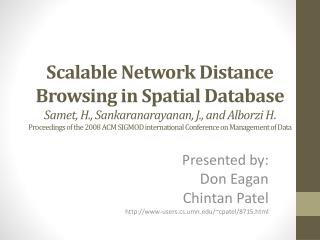DownloadDownload PresentationPresented by: Don Eagan Chintan Patel www-users.cs.umn/~cpatel/8715.html

# Presented by: Don Eagan Chintan Patel www-users.cs.umn/~cpatel/8715.html

Télécharger la présentation## Presented by: Don Eagan Chintan Patel www-users.cs.umn/~cpatel/8715.html

- - - - - - - - - - - - - - - - - - - - - - - - - - - E N D - - - - - - - - - - - - - - - - - - - - - - - - - - -
##### Presentation Transcript

1. Scalable Network Distance Browsing in Spatial DatabaseSamet, H., Sankaranarayanan, J., and Alborzi H. Proceedings of the 2008 ACM SIGMOD international Conference on Management of Data Presented by: Don Eagan Chintan Patel http://www-users.cs.umn.edu/~cpatel/8715.html

2. Outline • Motivation • Problem Statement • Proposed Approach • Other Approaches • Evaluation • Our Comments • Questions

3. Motivation • Growing Popularity of Online Mapping Services

4. Motivation • Real Time Shortest Paths

5. Motivation • Static Network, Variable Queries • Find Gas Stations, Hotels, Markets etc.

6. Motivation • Static Network, Variable Queries • Find Gas Stations, Hotels, Markets etc.

7. Problem Statement • Input: • Spatial Network S, Node q from S • Output: • k-nearest neighbors of q • Objective: • Facilitate “fast” shortest path queries based on different search criteria's • Constraints/ Assumptions: • Static spatial network • Contiguous (connected) regions

8. Challenges • Real-time response • Calculating all pairs shortest path is costly • Storing pre-computed naïvely doesn’t solve the problem • Scalability

9. Contribution • Efficient path encoding • Efficient retrieval • Abstracting shortest path calculation from domain queries

10. Key Concepts • Spatial Networks • Nearest Network Neighbor • Quad Tree • Morton Blocks • Decoupling • Scalability • Pre-computing

11. Spatial Networks • Graph with spatial components represented as nodes/ edges • Most Transportations are modeled as graph • Intersection – Node/ vertex • Roads – Edge • Time/ Distance – Edge Weight

12. K-Nearest Neighbors

13. K-Nearest Neighbors

14. K-Nearest Neighbors

15. K-Nearest Neighbors

16. K-Nearest Neighbors

17. K-Nearest Neighbors

18. Shortest Path • Dijkstra’s algorithm • Doesn’t work for real-time queries • Computationally expensive

19. Proposed Approach • Pre-compute shortest paths • Store and Retrieve Efficiently N = Number of vertices, M = Number of edges, s = Length of the shortest path

20. Path Encoding • Path coherence • Vertices in close proximity share portion of the shortest paths to them from distant sources

21. Path Encoding • Path coherence • Vertices in close proximity share portion of the shortest paths to them from distant sources

22. Path Encoding • Path coherence • Vertices in close proximity share portion of the shortest paths to them from distant sources

23. Path Encoding • Path coherence • Vertices in close proximity share portion of the shortest paths to them from distant sources

24. Path Encoding • Quadtree: Decompose until all vertices in block have same color

25. How is space reduced? • Capturing boundaries !

26. Path Retrieval • Retrieve quadtree corresponding to s

27. Path Retrieval • Find connected node t in the quadtree containing d

28. Path Retrieval • Repeat the process

29. K-nearest Neighbor • Set of objects • Pre-computed paths (quadtree)

30. K-nearest Neighbor • K = 2

31. K-nearest Neighbor • Queue1: m a b • Queue2: a b

32. K-nearest Neighbor • Queue1: a g e b f • Queue2: a g

33. K-nearest Neighbor • Queue1: a g e • Queue2: a g

34. K-nearest Neighbor • Return a and g

35. Other Approaches • IER: Incremental Euclidian Restriction • Based on Euclidian distance • Dijkstra’s algorithm to get network distance • INE: Incremental. Network Expansion • Dijkstra'salgorithm with a buffer L containing the k nearest neighbors seen so far in terms of network distance

36. Evaluation • Micro benchmark • Synthetic Data

37. Evaluation • Real Data Set: Major Road of the USA

38. Our Comments • We Liked: • Decoupling shortest path and neighbor calculation • Space reduction approach • Scalable • Correctness proofs • Detailed discussion about KNN variants

39. Our Comments • What we didn’t like: • Experiments: • No comparison with other approaches (e.g. hierarchical, dynamic etc.) • No performance graphs/ discussion with real dataset

40. Discussion • Other use cases? • Real Application: How to overcome assumptions?

41. Questions ? ? ?